The SURVEYLOGISTIC Procedure

### Link Functions and the Corresponding Distributions

Four link functions are available in the SURVEYLOGISTIC  procedure. The logit function is the default. To specify a different link function, use the LINK= option in the MODEL statement. The link functions and the corresponding distributions are as follows:

• The logit function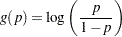is the inverse of the cumulative logistic distribution function, which is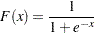• The probit (or normit) function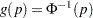is the inverse of the cumulative standard normal distribution function, which is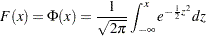Traditionally, the probit function includes an additive constant 5, but throughout PROC SURVEYLOGISTIC, the terms probit and normit are used interchangeably, previously defined as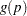.

• The complementary log-log function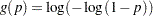is the inverse of the cumulative extreme-value function (also called the Gompertz distribution), which is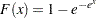• The generalized logit function extends the binary logit link to a vector of levels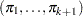by contrasting each level with a fixed level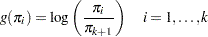The variances of the normal, logistic, and extreme-value distributions are not the same. Their respective means and variances are

Distribution

Mean

Variance

Normal

0

1

Logistic

0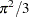Extreme-value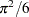where
is the Euler constant. In comparing parameter estimates that use different link functions, you need to take into account the different scalings of the corresponding distributions and, for the complementary log-log function, a possible shift in location. For example, if the fitted probabilities are in the neighborhood of 0.1 to 0.9, then the parameter estimates from using the logit link function should be about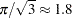larger than the estimates from the probit link function.Previous Page | Next Page | Top of Page